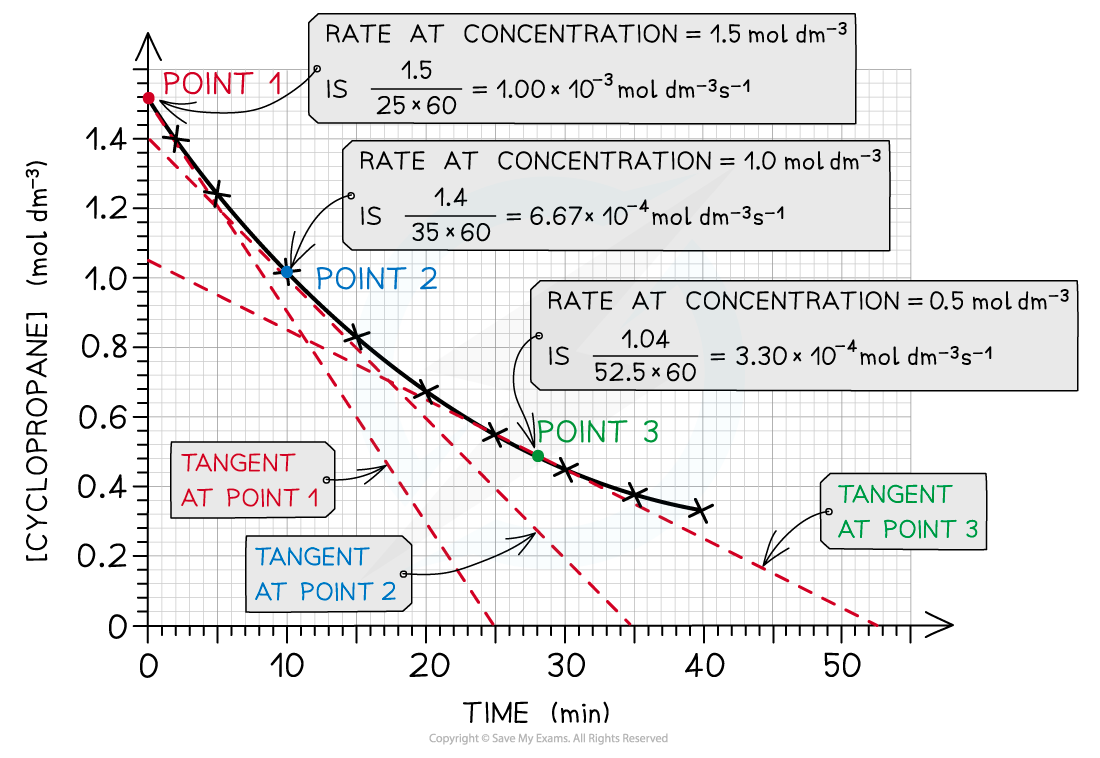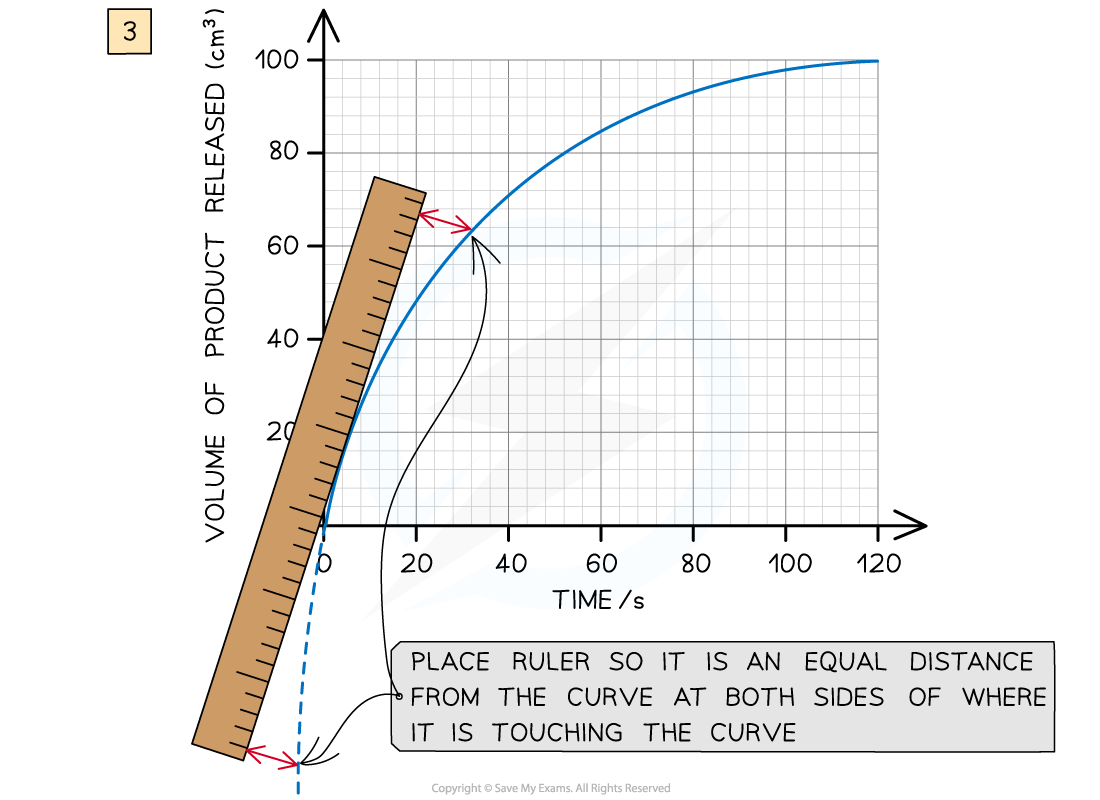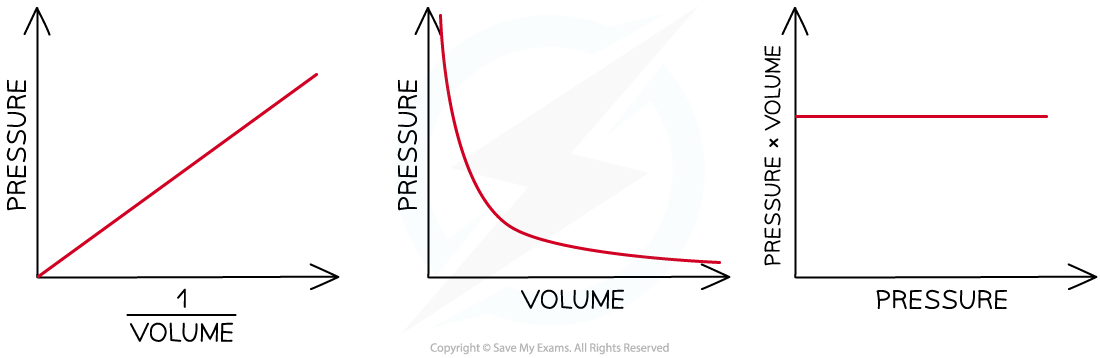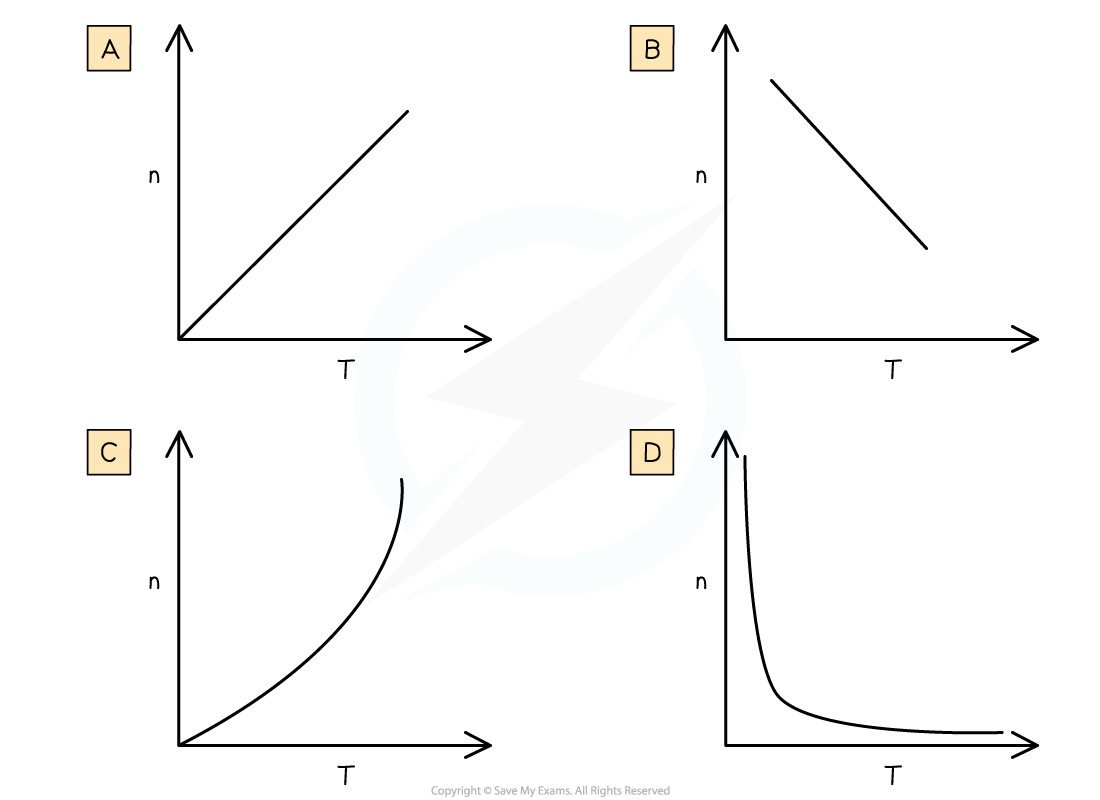# IB DP Chemistry: SL复习笔记11.2.6 Analysing Graphs

### Analysing Graphs

• The gradient of a graph can be found by:
• In the case of a straight line graph: using a triangle and the equation for a straight line
• In the case of a curve: drawing a tangent to the graph
• The triangle should be as large as possible to minimise precision errors
• The equation for a straight line is y = mx + c, where:
• y = dependent variable
• x = independent variable
• m = slope
• c = y-intercept
• The gradient or slope is therefore : m = ∆y/∆x
• This example from Kinetics illustrates the calculation of rates from a curveThe gradient can be found at different points on a curve. Here it has been multiplied by 60 to convert it from minutes-1 to seconds-1

• In the case of curves you will need a ruler to line up against the curve at the point you want to measure the gradient:Lining up a ruler against the curve is essential to drawing a tangent accurately

#### Exam Tip

Be careful that you process the units correctly when finding the gradient. The gradient unit is the y-unit divided by the x-unit, so in the example above the gradient of the curve is measured in cms-1

### Sketched Graphs

• Sketched graphs are a way to represent qualitative trends where the variables shown are often proportional or inversely proportional
• Sketched graphs do not have scales or data points, but they must have labels as these examples from the Gas Laws show:Sketched graphs show relationships between variables

### Graphical Relationships

• In the first sketch graph above you can see that the relationship is a straight line going through the origin
• This means as you double one variable the other variable also doubles so we say the independent variable is directly proportional to the dependent variable
• The second sketched graph shows a shallow curve which is the characteristic shape when two variables have an inversely proportional relationship
• The third sketched graph shows a straight horizontal line, meaning as the independent variable (x-axis) increases the dependent variable does not change or is constant

#### Worked Example

Which graph shows the correct relationship between the number of moles of a gas, n, and the temperature, T, at constant pressure and volume?The correct option is D

• The Ideal Gas Equation is PV= nRT.
• If P, V and R are constant then PV/R = nT = a constant
• n must be inversely proportional to T, which gives graph D